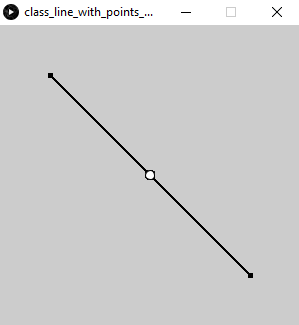# Processing.py: How to calculate the midpoint of a line

To calculate the midpoint of a line, use the following formula to calculate the x and y midpoints:

```# Middle of line
# midpoint = (xM, yM)
xM = (x1 + x2)/2
yM = (y1 + y2)/2```

Then the midpoint coordinates `(xM, yM)` can be used to draw an ellipse on the line. For example:

`ellipse(xM, yM, 10, 10)`

Here is a more complete code example, showing this in practice:

```x1 = 50
y1 = 50
x2 = 250
y2 = 250

# circle_size
cs = 4

def setup():
size(300, 300)
stroke(0)

def draw():
fill(0)
l1 = line_with_points(x1, y1, x2, y2, cs)

class line_with_points(object):
def __init__(self, x1, y1, x2, y2, cs):
self.x1 = x1
self.x2 = x2
self.y1 = y1
self.y2 = y2
self.cs = cs

# Beginning of line
ellipse(x1, y1, cs, cs)
# End of line
ellipse(x2, y2, cs, cs)
line(x1, y1, x2, y2)

# Middle of line
xM = (x1 + x2)/2
yM = (y1 + y2)/2
fill(255)
ellipse(xM, yM, cs+5, cs+5)```

Which produces: